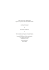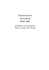# TechMAP Sample

## Transcription

TechMAP Sample
```TechMAP Sampler
TechMAP is a new NSF-funded project that has developed high school modules in
technical applications of mathematics.
The mathematics required by state tests is taught in algebra and geometry courses, but for
many students this is not enough. TechMAP modules demonstrate these skills in
vocational fields; they help reinforce existing mathematics courses through actual career
activities. These modules are self-contained with exercises, teacher notes, handouts, etc.,
and can be used individually or together. Each module takes one to three weeks of
classroom time.
Enclosed in this sampler you will find:
•
•
•
•
Matrix of TechMAP modules, including module title, mathematics course, and
mathematics topic.
Lesson 1 of Data, Data, Everywhere including teacher notes as well as the
Lesson 1 of The Right Package including teacher notes as well as the answers to
this lesson.
TechMAP order form.
TechMAP Modules
Module Title
Software
Mathematics Course
Mathematics Topic
Bracing Grids
Geometry
Discrete Math
Pythagorean Theorem
Graph Theory
Plan the Work, Then Work the
Plan
Discrete Math
Scheduling
Bin Packing
On the Botpath*
X
Geometry
Algebra I & II
Coordinates, Slope
Systems of Equations
Where Shall I Sell My Wheat?
X
Algebra II
Precalculus
Conics (hyperbola)
GPS
Geometry
Sphere
Latitude & Longitude
Sound
Algebra II
Exponents
Logarithms
Geometry
Surface Area
Volume, Measurement
Algebra II
Exponential Functions
Growth & Decay
Geometry
Circles
(central & inscribed
angles)
Insulation
Algebra II
Logarithms, Equations,
Linear Regression
Clocks
Algebra II
Direct Variation
Indirect Variation
Strength of Beams
Algebra I
Direct Variation
Indirect Variation
Formulas for Success*
Algebra I
Expressions
Equations
The Right Size*
Geometry
Measurement
The Right Package*
X
Tim and Tom's Financial
Circle Relationships in
Carpentry
X
What Are the Chances?*
Probability & Statistics
Probability: Basic, Joint,
Conditional
Line Up*
Algebra I
Statistics
Linear Relationships
Similarity *
Geometry
Similar Figures
(sides, areas, volumes)
What's Next?*
Algebra I & II
Sequences
Exponential Functions
Data, Data, Everywhere*
Algebra I, Statistics
Statistical Graphs
Averages
Over the Limit*
Algebra I & II
Inequalities
Circular Motion
Algebra II
Precalculus
Trig Functions
Finding the Right Triangle
Geometry
Algebra I & II
Right Triangle
Trigonometry
*Denotes modules targeted at high-stakes tests. Each of these modules includes a
supplemental section of problems taken from the MCAS or other state tests. These
supplemental problems are related to the mathematical content of the module.
TechMAP
DATA, DATA, EVERYWHERE
TEACHER EDITION
1
0
0
4
9
3
3
7
3
9
0
2
4
8
1
7
3
4
1
5
8
2
8
3
5
5
1
6
3
1
5
8
2
7
1
4
6
5
3
7
8
1
5
2
3
7
1
8
4
0
6
4
8
1
4
0
1
6
3
1
0
9
5
2
2
6
8
2
1
9
3
8
1
4
9
3
1
1
0
0
1
1
1
0
0
0
DATA, DATA, EVERYWHERE
TEACHER EDITION
by COMAP, Inc.
The Consortium for Mathematics and Its Applications (COMAP)
57 Bedford Street, Suite 210
Lexington, MA 02420
or transmitted in any form or by any means, electronic or mechanical, including
photocopying, recording, storage in an information retrieval system, or otherwise,
without prior written permission of the publisher.
Data, Data, Everywhere
1
0
0
4
9
3
3
7
3
9
0
2
4
8
1
7
3
4
1
5
8
2
8
3
5
5
1
6
3
1
5
8
2
7
1
4
6
5
3
7
8
1
5
2
3
7
1
8
4
0
6
4
8
1
4
0
1
6
3
1
0
9
5
2
2
6
8
2
Marsha Davis
1
9
3
8
1
4
9
3
1
1
0
0
1
1
1
0
0
0
Data, Data, Everywhere
D
ata are collected every day. Whether you know it or not, you contribute to
the amount of data. Every time you make a phone call, the number,
location, date, and length of the call are recorded. When you rent movies
at your local video store, the titles, dates rented, dates returned, and fines are
saved in the store’s computer. When you use a store card to get discounts, the
date, products you buy, and amount you spend are stored in a data bank. At the
reference.
The situation with data is much like the situation in Samuel Taylor Coleridge’s
poem The Rime of the Ancient Mariner. The ancient mariner (old sailor), stuck in
the middle of an ocean and very thirsty, exclaims:
Water, water, everywhere,
Nor any drop to drink.
Sometimes, we are stuck in a sea of data and still thirsty for information. For
example, look at the data in Figure 1. These numbers are neatly organized in
columns and rows. However, they don’t tell you much.
4
3
5
8
1
6
1
5
2
3
5
4
4
4
7
7
1
8
5
5
3
1
5
1
3
2
5
2
5
5
4
3
7
8
8
8
4
7
5
1
6
6
8
1
3
7
6
7
1
1
1
6
1
8
5
8
1
3
5
8
2
8
7
4
4
6
5
1
1
7
7
3
1
5
1
7
5
5
1
5
1
5
1
6
3
2
1
5
6
1
5
5
5
1
6
6
5
2
8
4
8
6
1
3
5
1
1
1
1
6
2
1
7
5
1
7
7
6
1
7
3
5
8
3
2
6
6
3
1
1
2
8
1
4
2
3
3
3
5
6
2
2
4
5
6
6
5
1
8
2
1
5
5
3
1
5
2
8
1
7
1
4
5
5
7
Figure 1.
Data consisting of 165 numbers.
Before you can make sense of these data, you need to know what they represent.
Then you need to process the numbers in order to extract useful information.
The data in Figure 1 are 165 responses from 12th-grade students to the
question:
“On the average over the school year, how many hours per week do you work in
a paid or unpaid job?”
2
TechMAP Data, Data, Everywhere
Module Opener
Each student picked one of the following choices:
(1) None
(2) 5 or fewer hours
(3) 6 to 10 hours
(4) 11 to 15 hours
(5) 16 to 20 hours
(6) 21 to 25 hours
(7) 26 to 30 hours
(8) More than 30 hours
Now you know what the numbers in Figure 1 represent. However, what do they
tell you about student work patterns? If you have trouble answering this
students. This question and the data in Figure 1 are part of the ongoing study
Monitoring the Future: A Continuing Study of American Youth.
In this module you will learn techniques for organizing, summarizing, and
from data.
TechMAP Data, Data, Everywhere
Module Opener
3
LESSON 1
Frequency
Tables
Category Data
Graphical
Displays
Data come in two basic types—category data and numeric data. The
techniques you use to analyze data depend on the type. In this lesson you will
learn how to analyze category data.
Activity 1
Exercise Set 1
Category data describe a characteristic of the items in the sample. For
example, if you describe cars by color (red, white, silver, black, other), the result
is category data. Other examples of category data are pizza toppings (cheese,
pepperoni, veggie), hair color (red, blonde, brunette), and types of wood (oak,
cedar, pine).
Numeric data are numbers that have meaning in terms of size. For example, if
you measure the heights of the students in your class, the data are numeric. Just
because data are numbers does not make them numeric data. For example, the
data in Figure 1 of the module opener are numbers. However, each of the
numbers represents a category (1 = “None,” 2 = “5 or fewer hours,” 3 = “6 to 10
hours,” and so forth). Hence, the data in Figure 1 are category data.
FREQUENCY TABLES
Look again at Figure 1, which gives student responses to the question:
“On the average over the school year, how many hours per week do you work in
a paid or unpaid job?”
It is difficult to learn anything from the 165 numbers. However, you can learn a
lot when the data are organized into the frequency table in Figure 2.
Category
Frequency
Percent (%)
(number of data in category)
(1) None
38
23.0
(2) 5 or fewer hours
14
8.5
(3) 6 to 10 hours
17
10.3
(4) 11 to 15 hours
12
7.3
(5) 16 to 20 hours
34
20.6
(6) 21 to 25 hours
18
10.9
(7) 26 to 30 hours
16
9.7
(8) More than 30 hours
16
9.7
165
100.0
Total
4
TechMAP Data, Data, Everywhere
Figure 2.
A frequency table.
Here’s how to make this table.
■
■
■
The first column contains a list of the possible data values
(categories).
The second column shows the number of times (frequency)
each data value occurs. For example, you find thirty-eight
1s, fourteen 2s, etc. in Figure 1.
The third column gives the percentage (rounded to the
nearest tenth of a percent). For example, the percentage for
category 1 is calculated as follows:  38  × 100% ≈ 23.0%.
 165 
To calculate the percentage, divide the frequency by the total number

frequency

of data and multiply the result by 100%:  total number of data × 100%
■
The last row of the table gives the totals. The total for the
second column is the sum of the numbers in column 2. This
tells you the number of data. The total for the third column
is the sum of the percents in column 3. This total should be
100%. (Sometimes this total is slightly less or more than
100% due to roundoff.)
Finally, the data are in a form that gives you information about students’
work patterns. You now know that 23.0% of the students do not work at any
job during the school year (category 1). That is the highest percent for any of
the categories. However, category 5 (students who worked between 16 and 20
hours per week) has the next highest frequency. The 5s account for 20.6% of
the responses.
GRAPHICAL DISPLAYS
You’ve heard the saying “A picture is worth a thousand words.” Sometimes it’s
easier to understand information if it is presented in the form of a picture or
chart. Bar charts and pie charts are the most common displays for category data.
IN THE NEWS …
LOS ANGELES, June 16, 2003 — While there are a variety of
reasons contributing to the downturn in music sales, the two problems
according to a recent national survey of 12 to 44 year olds conducted
music from the Internet for playback at another time. The graph below
TechMAP Data, Data, Everywhere
5
Percent
50
40
30
20
10
0
51 to 99 100 or more
20 or fewer 21 to 50
example, the tallest bar in the chart is above the category “100 or more.” That
The information on students’ work patterns in Figure 2 could also be displayed
in a bar chart. Here are the directions on how to make a bar chart:
Creating a Bar Chart
Step 1: Draw a horizontal axis and a vertical axis.
Step 2: Count the number of categories. Mark evenly-spaced
tick marks on the horizontal axis—one tick mark for each
category. Label them with the category names.
Step 3: Scale the vertical axis for frequency (or percent). Your
tick marks on this scale should be equally-spaced. The
numbers next to these tick marks should jump by the same
amount as you move from one tick mark to the next.
Step 4: Centered over each category on the horizontal axis,
draw a rectangle (bar). The rectangle’s height should equal
the frequency (or percent) of the category. The bars should
have the same width. They should not touch each other.
Step 5: Label the axes and give the graph a title.
Figures 4–8 show you how to apply these five steps to make a bar chart for the
students’ work data from Figure 2.
Figure 4. Step 1: Draw the axes.
6
TechMAP Data, Data, Everywhere
25
20
15
10
Figure 5. Step 2: Mark categories on the horizontal axis.
more than 30 hours
26 to 30 hours
21 to 25 hours
16 to 20 hours
11 to 15 hours
6 to 10 hours
5 or fewer hours
none
more than 30 hours
26 to 30 hours
21 to 25 hours
16 to 20 hours
11 to 15 hours
6 to 10 hours
5 or fewer hours
none
5
Figure 6. Step 3: Add a percent scale to the vertical axis.
Hours Worked Per Week During School Year
25
20
20
Percent
25
15
10
15
10
26 to 30 hours
more than 30 hours
Hours Worked
21 to 25 hours
16 to 20 hours
11 to 15 hours
6 to 10 hours
5 or fewer hours
more than 30 hours
26 to 30 hours
21 to 25 hours
16 to 20 hours
11 to 15 hours
6 to 10 hours
5 or fewer hours
none
Figure 7. Step 4: Draw the bars.
none
5
5
Figure 8. Step 5: Label axes and add title.
Notice from Figure 8 that roughly half as many students work 6 to 10 hours as
16 to 20 hours. That’s because the bar over the category “6 to 10 hours” is
about half the height of the bar over the category “16 to 20 hours.”
In the examples shown, the horizontal axis is used for the category and the
vertical axis for the frequency or percent. Sometimes people get creative in their
bar charts. They might switch the axes and put the categories on the vertical
axis. Also, they might give the bars characteristics of the topic. The newspaper
clipping in Figure 9 is a great example.
TechMAP Data, Data, Everywhere
7
IN THE NEWS …
A breath of fresh air
Two thirds of homes have some type of air conditioning, accounting
for 14% of residential electric expenditures.
Air Conditioning in USA homes
44%
Central air
Window units
None
25%
Figure 9.
32%
A pie chart is another way to display category data. A pie chart is a circle
divided into slices. Each slice represents a category. The pie chart in Figure 10
is based on data from the music survey conducted by Edison Media Research.
(See the In the News segment near Figure 3.) The survey asked:
free from the Internet?”
The responses are represented by the pie chart in Figure 10.
Responses to Question
Responses to Question
No
40%
No
40%
144°
216°
Yes
60%
Figure 10. Pie chart
Yes
60%
Figure 11. Angles of the slices.
Drawing a pie chart by hand requires a protractor to measure angles. Figure 11
shows the angles of the slices. These angles are computed by multiplying the
angle of a full pie, 360°, by the percentage for the slice:
angle for the Yes slice is 60% of 360°: 0.60 × 360° = 216°.
angle for the No slice is 40% of 360°: 0.40 × 360° = 144°.
8
TechMAP Data, Data, Everywhere
Computer software such as Excel is often used to make the pie charts. What is
important is to be able to look at a pie chart and estimate the percentage in each
category from the size of the slice. Figure 12 displays three percents that can
50% is
50
100
=
1
2
of the pie (180°).
25% is
25
100
=
1
4
of the pie (90°).
12.5% is
1
8
of the pie (45°).
25%
50%
180°
90°
Figure 12. Slices representing 50%, 25% and 12.5%.
45°
12.5%
12.5%
Now that you have learned how to make frequency tables, bar charts, and pie
charts, you are ready to analyze data in Activity 1.
TechMAP Data, Data, Everywhere
9
Activity 1
In this activity, you will investigate more data from Monitoring the Future: A
Continuing Study of American Youth. You will focus on the four questions in
Figure 13. Data from 50 students who said their present high school program
was “vocational, technical, or commercial” are in Figure 14.
Question/ Response
1. Gender
What is your gender? Male, Female
2. Work
On the average over the school year, how many hours per week do you work in a paid
or unpaid job?
1 = “None”, 2 = “5 or fewer hours”, 3 = “6 to 10 hours”, 4 = “11 to 15 hours”, 5 = “16
to 20 hours”, 6 = “21 to 25 hours”, 7 = “26 to 30 hours”, 8 = “More than 30 hours”
3. Drive
During the average week, how much do you usually drive a car, truck, or motorcycle?
1 = “Not at all”, 2 = “1–10 miles”, 3 = “11–50 miles”, 4 = “51–100 miles”,
5 = “100 to 200 miles” and 6 = “More than 200 miles”
4. Ticket
Within the LAST 12 MONTHS, have you received a ticket (OR been stopped and
warned) for moving violations, such as speeding, running a stop light, or improper
passing?
Figure 13.
Questions and possible responses.
Yes, No
Gender
Work
Drive
Ticket
Gender
Work
Drive
Ticket
Gender
Work
Drive
Ticket
Female
5
4
No
Female
1
5
No
Male
8
1
No
Female
1
4
No
Male
4
4
No
Male
7
1
No
Male
5
1
No
Male
4
4
No
Male
5
6
Yes
Female
5
5
Yes
Female
6
5
No
Male
3
5
No
Male
8
3
No
Male
1
6
No
Female
6
5
Yes
Male
3
5
Yes
Male
7
6
No
Male
6
5
Yes
Male
6
5
Yes
Male
6
5
Yes
Male
4
1
No
Female
8
1
No
Female
1
1
No
Male
7
6
No
Female
7
5
No
Male
8
4
No
Male
8
5
Yes
Female
7
5
No
Male
1
3
No
Male
8
5
No
Male
1
1
No
Male
5
6
Yes
Female
3
1
No
Male
6
6
No
Female
1
2
No
Female
5
6
No
Male
3
4
No
Male
4
3
Yes
Female
5
6
No
Male
5
1
No
Female
8
4
Yes
Female
8
3
No
Male
8
5
No
Female
5
1
No
Male
5
4
No
Female
7
3
No
Male
7
5
Yes
Female
2
3
No
Male
1
6
Yes
Male
6
3
Yes
Figure 14. Data from 50 students in vocational, technical, or commercial programs.
10
TechMAP Data, Data, Everywhere
Activity 1
1. First, consider the data from the question on gender.
a) What percentage of the students are male? Explain your calculations.
b) What percentage of the students are female? Explain how you got your
c) Which of these pie charts represents your results from (a) and (b)?
Pie Chart for Gender
Pie Chart for Gender
Female
Female
B.
A.
Male
Male
Pie Chart for Gender
Pie Chart for Gender
Male
Male
D.
C.
Female
Female
2. Next, consider the data on the number of hours that the students work.
a) Make a frequency table for the hours students work. Make it similar to
Figure 2.
Your frequency table in (a) is based on a sample of 50 students attending
vocational, technical, or commercial programs. The frequency table in
Figure 2 is based on a sample of 165 high school students (all types of
programs are included).
b) Do the work patterns of students in vocational, technical, or commercial
programs appear to be different from the work patterns of high school
students in general? Explain. Use numbers from both tables (your table
from (a) and the table in Figure 2) in your explanation.
c) Draw a bar chart that represents the information in your frequency table
in (a).
TechMAP Data, Data, Everywhere
Activity 1
11
The next two questions focus on driving habits.
3. a) What percentage of students do not drive cars, trucks, or motorcycles
during an average week?
b) What percentage of students do drive cars, trucks, or motorcycles during
an average week?
c) Make a pie chart that represents the information from (a) and (b).
Explain how you determined the angles of the slices.
4. Focus only on the students who drive during an average week (categories
2–6).
a) How many of the students in this sample drive during an average week?
b) Of the students who drive during an average week, what percentage gets
a ticket or warning? Explain how you got your answer. Show your
calculations.
c) Based on your answer to (b), do you think that police target high school
12
TechMAP Data, Data, Everywhere
Activity 1
Exercise Set 1
1. The bar chart in Figure 15 is based on data from the 2003 National
Number of Music CDs Purchased
By Teens Ages 12 to 17
50
Percent
40
30
20
Figure 15.
Number of CDs bought by teens.
10
16 or more
11 to 15
6 to 10
1 to 5
0
Number of CDs Purchased in Last 12 Months
a) Estimate the percentage of teens that bought 16 or more CDs.
b) Estimate the percentage of teens that bought 1 to 5 CDs.
c) Which of the following is closest to the percentage of teens that bought
6 or more CDs?
A. 25%
B. 23%
C. 47%
D. 68%
d) Suppose the bar chart was based on data from 1500 teens.
About how many teens do you think bought 6 or more
CDs in the 12-month period? Show how you got your
2. Participants in the 2003 National Record Buyers Study
were asked to respond to the following statement: “You are
less passionate about music these days than you used to be.”
The results are displayed in Figure 16.
Response to Music Survey
Agree
Neither
Disagree
Figure 16. Response to a statement.
TechMAP Data, Data, Everywhere
Exercise Set 1
13
Which of the following tables best represents the information in the pie
A.
Response
Percent
Agree
30
Disagree
71
Neither
2
Response
Percent
B.
Agree
40
Disagree
50
Neither
2
Response
Percent
C.
Agree
25
Disagree
73
Neither
2
Response
Percent
D.
Agree
41
Disagree
58
Neither
1
3. As part of the 2002 survey from Monitoring the Future: A Continuing Study of
think you are compared to others your age? The frequency table in Figure
17 shows the results.
Response
14
Frequency
Far below average
121
Below average
745
Average
4102
Above average
6679
Far above average
1048
Percent
TechMAP Data, Data, Everywhere
Figure 17.
Results on the intelligence question.
Exercise Set 1
a) How many students answered this question?
b) Copy the table in Figure 17. Fill in the percent column with the
of a percent.
c) Represent the categories and percents from (b) in a bar chart.
4. Refer to the bar chart on air conditioning in Figure 9. The caption reads
that two thirds of homes have some type of air conditioning. Do you agree
with this statement? Explain.
1993–1995 Market
Projections for Salons’
Top Nail Services
(Billions of dollars)
4
A survey of U.S. nail
technicians indicates
consumers spend far more
on artificial nails than on
manicures. Spending on
artificial nails in U.S. salons
rose from just under \$3
billion in 1993 to more than
\$4 billion in 1995, while
spending on manicures
remained under \$1 billion
during the same period.
3
2
1
0
Figure 18.
Bar chart for nail services 1993–1995.
1994 1995
1993
Artificial nails
and extensions
1993 1994 1995
Manicures
5. The bar chart in Figure 18 shows the amounts that consumers spent on
artificial nails/extensions and manicures in 1993, 1994, and 1995.
a) In 1995 consumers spent an estimated \$5.2 billion on nail services.
Estimate the percent that was spent on artificial nails/extensions. (Round
your answer to the nearest tenth of a percent.) Explain how you got your
TechMAP Data, Data, Everywhere
Exercise Set 1
15
b) Which of the pie charts below best represents consumer spending
in 1995?
Consumer Spending on Nail Services
Consumer Spending on Nail Services
Artificial Nails/
Extensions
A.
Artificial Nails/
Extensions
B.
Manicures
Manicures
Consumer Spending on Nail Services
Consumer Spending on Nail Services
Artificial Nails/
Extensions
Artificial Nails/
Extensions
D.
C.
Manicures
Manicures
6. The bar chart in Figure 18 shows the amounts that consumers spent on
artificial nails/extensions and manicures in 1993, 1994, and 1995.
a) Measure the length of the bar (finger) that represents the amount spent
for artificial nails/extensions in 1993. Then measure the length of the bar
that represents the amount spent for manicures in 1993.
b) Based on your measurements, approximately how many times as
much was spent on artificial nails/extensions as on manicures?
7. The pie chart in Figure 19 represents grades given in a math course.
a) Which of the following is closest to the percent of students who got
a B in the course?
A. 120%
B. 45%
C. 33%
B
A
120°
60°
180°
C
D. 22%
Figure 19. Pie chart of grades in a math course.
16
TechMAP Data, Data, Everywhere
Exercise Set 1
b) Approximately what percentage of the class got As?
c) Approximately what percentage of the class did not get As?
8. Figure 20 shows the recommended percentages of protein, fat, and
carbohydrates for one diet plan.
Recommended Diet
Carbohydrates
55%
Protein
Fat
30%
Figure 20.
Pie chart of protein, fat, and carbohydrates.
a) According to these recommendations, what percentage of your diet
should be protein?
b) Represent the same information in a bar chart.
TechMAP Data, Data, Everywhere
Exercise Set 1
17
Data, Data, Everywhere: Teacher Notes
A Note on Context
This module investigates the statistical techniques for extracting information from
data. We live in a world of data. There are databases with information about the food
we buy, videos we rent, phone calls we make, and tollbooths we drive through.
When data are collected and used properly, displays and statistics based on the data
can provide useful information, which can guide decisions.
Topics of the data contained in this module are varied. They include work patterns
career home runs for players with all-time top batting averages, daily pulse rates and
blood pressure readings for nursing home patients, and treadwear ratings for tires.
The module introduces students to techniques for analyzing both category and
numeric data. Frequency tables, bar charts, and pie charts are used to analyze
category data. Numeric data are displayed with stem-and-leaf plots, line plots,
histograms, and box plots. In addition, students learn how to calculate basic
statistics such as mean, median, mode, and range. These techniques help unlock the
information contained in the data so the data can tell their story.
Technology Note
Graphing calculators are recommended for use throughout this module. In Lesson 3
in particular, students should be encouraged to use graphing calculators to calculate
statistics and display data. Since graphing calculators are allowed on many
standardized exams, student scores may improve if students know how to use them.
Module Opener
The purpose of this opening reading is to convince students that a lot of data by
itself provides little information. For example, the numbers in Figure 1 look more
like something out of The Matrix movies rather than information on the work
The data from Figure 1 are a small subset of the data collected for the study
Monitoring the Future: A Continuing Study of American Youth (12th-Grade Survey),
2002. The 2002 study is part of an annual series. The series is designed to document
changes in important values, behaviors, and lifestyles of American youth. More
information can be found at the National Archive of Criminal Justice Data, where
it is cataloged as study number 3753. The URL is
http://webapp.icpsr.umich.edu/cocoon/NACJD-STUDY/03753.xml
TechMAP
Data, Data, Everywhere
Teacher Notes 1
Lesson 1
Materials List
Protractors
Rulers (Exercise 6)
The purpose of this reading is to give students background in analyzing category
data. The techniques introduced include frequency tables, bar charts, and pie charts.
It may be helpful if students check for themselves the frequencies in Figure 2.
Have different groups of students count the 1s, 2s, 3s, 4s, 5s, 6s, 7s and 8s in Figure
1.
This would also be a good time to review how to compute percentages. Students
should use calculators for the division. However, you may want to remind students
that when you multiply a number by 100, you need only move the decimal point
two places to the right. Stress some equivalences between some common fractions
and percentages. For example, 1/2 is equivalent to 50%, 1/4 to 25%, 3/4 to 75%,
and 1/3 to 33.3%. Unless students are told to round to the nearest percent,
encourage students to retain one decimal when writing percents.
The In the News segment is from the National Record Buyers Study III, conducted
by Edison Media Research. The complete report (filled with a variety of bar charts
and pie charts) can be found at the following URL:
In this lesson students learn how to make frequency tables, bar charts, and pie
charts for category data. If you want students to draw a pie chart by hand, you will
need to provide protractors. Some state exams provide protractors and rulers during
the exam. However, for most questions students need only approximate the correct
size of a pie slice. Hence they should be familiar with basic percentages: 50% is
half of the pie, 33.3% is one-third of the pie; 25% is one-fourth of the pie; 12.5%
is one-eighth of the pie.
Activity 1
The purpose of this activity is to give students an opportunity to practice what they
have learned on a set of real data from the study Monitoring the Future: A Continuing
Study of American Youth. These data are from students who identified their high
school program as vocational, technical, or commercial. (The data from Figure 1
included high school students enrolled in all types of programs.) The activity
includes data from four questions on gender, work, and driving.
Students could use protractors to answer Question 1, which involves pie charts.
However, giving students an opportunity to reason what the correct size of the slice
should look like is probably more beneficial. The correct angle for the slice
representing the Males should be 62% of 360° or 223.2°. However, 62% is between
2 Teacher Notes
Data, Data, Everywhere
TechMAP
50% and 75%. Hence, the slice representing 62% should be between one-half and
three-quarters of a pie.
Make sure in Question 2(b) that students compare percents and not frequencies.
This is an open response question. Many state exit exams contain open response
questions and students need practice answering them. Set aside some time to
discuss student answers to this question.
Question 3 would be a good place for students to use protractors.
Exercise Set 1
The purpose of this set of exercises is to provide additional practice with frequency
tables, bar charts, and pie charts. Some of the questions are patterned after
questions that appear on state exit exams.
Exercise 1 is a multi-part question that focuses on estimating percents from a bar
chart. Part (d) asks students to calculate a percentage of a particular quantity.
Exercise 2 asks students to estimate percentages in each category given a pie chart.
Exercise 3 gives the frequencies and sample size and asks students to calculate the
percents. Then they draw a bar graph, which has a percent scale on the vertical
axis.
Exercise 4 asks students to reconcile a discrepancy between 68% and the fraction
2/3.
Exercises 5 and 6 are based on a rather elaborate bar graph, in which the bars are
drawn as fingers. In Exercise 5, students estimate a percentage from the bar graph
and then select a pie chart that represents that percentage. In Exercise 6, students
estimate how many more dollars are in one category than another by comparing the
height of the bars (fingers). They will need rulers for this exercise.
Exercises 7 and 8 are based on pie charts. In Exercise 7, students estimate
percentages from a pie chart. In Exercise 8, they draw a bar chart that represents
the same information contained in a pie chart.
TechMAP
Data, Data, Everywhere
Teacher Notes 3
Lesson 1
Activity 1
1. a) There were 31 males out of 50 students.
Percent of males = (31/50) × 100% = 62.0%.
b) Percentage of females = 100% – 62% = 38%; or, (19/50) × 100% = 38.0%.
c) C ; Sample explanation: Pie chart A shows more females than males. Pie
chart B shows equal numbers of males and females. Since there are more
males than females in the sample, both A and B should be rejected. In D,
the slice representing the females appears to be roughly 1/4 th or 25% of
the pie. This percentage is too low. Hence, by a process of elimination, the
correct answer is C. Furthermore, the slice of pie C that represents the
males is larger than the slice in B and smaller than the slice in D; hence, the
slice for males in pie C represents between 50% and 75%. This fits in with
the actual percentage of 62%.
2. a)
Category
Frequency
Percent (%)
(1) None
8
16
(2) 5 or fewer hours
1
2
(3) 6 to 10 hours
4
8
(4) 11 to 15 hours
4
8
(5) 16 to 20 hours
10
20
(6) 21 to 25 hours
7
14
(7) 26 to 30 hours
7
14
(8) More than 30 hours
9
18
50
100
Total
b) It does not make sense to compare frequencies since the sample size for the
frequency table in Figure 2 is 165 students and the sample size for the
frequency table from (a) is 50 students. In order to make a comparison, the
percentages must be used. This is an open response question and hence
Sample answer: In the frequency table from Figure 2, the highest percentage
was in the category of none: 23% of the high school students (all programs)
did not work during the school year. This percentage is lower for students
enrolled in a vocational, technical, or commercial (votechcom) program; only
16% of those students did not work during the school year. The highest
TechMAP
Data, Data, Everywhere
percentage for the votechcom students fell in the category of working 16 to
20 hours. This was the second highest percentage for the mixed high school
students (all programs). The two percentages, 20% for votechcom students
and 20.6% for the mixed high school students, are about the same. The
largest difference is in the percentages for students who work more than 20
hours per week: 46% for the votechcom students and only 30.3% for the
mixed high school students. In conclusion, a higher percentage of the mixed
students than the votechcom students did not work during the school year. A
considerably higher percentage of votechcom students than mixed students
worked 21 or more hours.
c)
Note: Students may choose a shorter title. They may also replace the
frequency scale with a percentage scale, in which case the numbers on the
vertical axis would double. However, the relative sizes of the bars to each
other should remain the same for all histograms, regardless of the scale
chosen for the vertical axis.
3. a) Ten students reported they did not drive at all. That is (10/50) × 100% =
20% of the students.
b) If 20% of the students did not drive at all, then 100% – 20% = 80% did drive
during an average week.
Data, Data, Everywhere
TechMAP
c) The angle for the slice representing the non-drivers is 20% of 360°:
(0.20)(360°) = 72°. Thus, the angle for the slice representing the drivers is
360° – 72° = 288°. The pie chart below is drawn using a protractor.
If students do not have protractors, they can approximate this pie chart by
first drawing a quarter slice (for 25%) and then making it a little smaller.
More precisely, they could divide the one-quarter slice into 5 equal slices and
remove one of these smaller slices as shown below:
The four small slices remaining will represent 20%.
4. a) 40
b) Sample answer: First, we crossed out all the students who answered 1 to
the Drive question. That left 40 students who answered 2–6, meaning they
drove more than one mile during an average week. From these remaining
students, we counted the number of Yes responses to the Ticket question.
There were 14 Yesses. The percentage of Yesses from the students who
drive is (14/40) × 100% = 35%.
c) This is a very open question and there are many good answers.
Sample answer: On the surface, it appears that police do target high school
students when they give out tickets or warnings because the percentage of
35% is very high. One possibility is that a high percentage of high school
students exceed the speed limit when driving, run stop signs or pass
improperly. Perhaps the percentage of high school students who engage in
these practices is higher than for drivers in general. Thus, high school
students are more likely to get stopped. Another possibility is that the
percentage of all drivers that get tickets or warnings in a single year is also
TechMAP
Data, Data, Everywhere
about 35%. Hence, data from another survey is needed. There should be
three types of questions on this survey: (1) a question on high school status
(high school student, out of high school), (2) a question asking if the person
frequently exceeds the speed limit, runs stop signs, or passes improperly, and
(3) the ticket question from Figure 13.
Exercise Set 1
1. a) Around 15%
b) Around 53%
c) C
d) Using the answer to (c) or 47%, 47% of 1500 is (0.47)(1500) = 705.
2. D; Sample explanation: A and B can be eliminated because the percentages do
not add up to 100. In C, the percentage for Agree is one-quarter of the pie.
However, the actual slice for Agree is more than a quarter of a pie. Hence C can
be eliminated. The remaining answer is D.
3. a) 12,695
b) Here are the calculations:
(121/12,695) × 100% ≈ .953%; (745/12,695) × 100% ≈ 5.868%;
(4,102/12,695) × 100% ≈ 32.312%; (6,679/12,695) × 100% ≈ 52.611%;
(1,048/12,695) × 100% ≈ 8.255%.
Response
Frequency
Percent
(1) Far below average
121
1.0
(2) Below average
745
5.9
(3) Average
4,102
32.3
(4) Above average
6,679
52.6
(5) Far above average
1,048
8.2
c)
Data, Data, Everywhere
TechMAP
4. Sample answer #1: According to the bar chart in Figure 9, 32% of homes have
no air conditioning. That means that 100% – 32% = 68% of the homes have
some sort of air conditioning. Two thirds is equivalent to (2/3) × 100% ≈ 66.7%.
If you round up to the nearest percent, you only get 67%, a little short of 68%.
Sample answer #2: For their caption, USA Today wanted a fraction with a small
denominator that was close to 67%—2/3 would work for that purpose.
5. a) Sample (answers may differ slightly): The longest finger represents the
amount spent on artificial nails/extensions. It represents approximately \$4.2
billion. This is (\$4.2 billion/\$5.2 billion) × 100% ≈ 80.8% of the total
amount that consumers spent on nail services in 1995.
b) B
6. b) (length of 1993 finger for artificial nails)/(length of 1993 finger for
manicures) ≈ 3. Hence, approximately three times as much was spent on
artificial nails/extensions as on manicures.
7. a) C
b) (60/360) × 100% ≈ 17%. (Answers may vary slightly.)
c) 100% – 17% = 83%. (Answers may vary slightly.)
8. a) 15%
b)
TechMAP
Data, Data, Everywhere
TechMAP
THE RIGHT PACKAGE
TEACHER EDITION
THE RIGHT PACKAGE
TEACHER EDITION
by COMAP, Inc.
The Consortium for Mathematics and Its Applications (COMAP)
57 Bedford Street, Suite 210
Lexington, MA 02420
or transmitted in any form or by any means, electronic or mechanical, including
photocopying, recording, storage in an information retrieval system, or otherwise,
without prior written permission of the publisher.
The Right Package
Gary Froelich
The Right Package
M
ost people do not get very far in a day without using a package. The tube
that holds your toothpaste is a package. So is the bottle that holds your
shampoo. And many people have a breakfast of cereal that comes in a
box and milk that comes in a carton.
Many jobs involve packaging. If you work in a supermarket, nearly every
product you handle is packaged. Sometimes a product is packaged more than
once. For example, soda is often sold in cans, which are packages. Twelve cans
are packaged in a paperboard container. And the customer might carry the 12pack home in a bag, which is another package.
Here are a few facts about packaging.
■
■
■
■
■
■
The packaging business contributes over a trillion dollars to
the American economy each year.
In the United States, the packaging industry employs over a
million people.
Beverage packaging alone is a huge business. In the United
States, over 150 billion beverage containers are sold
annually.
About 10 cents of every dollar spent in a grocery store pays
for packaging.
About a third of America’s garbage is packaging.
Most companies that use packaging are trying to use less.
For example, Sunoco says it has reduced the amount of
paperboard in its packages by 14%. This amounts to a
savings of about 2000 tons a year.
Figure 1. An employee at Royal Paper Box
prepares a die that will be used to cut a
paperboard container.
At http://www.royalpaperbox.com, you can take
a virtual tour of the Royal factory.
Since packaging is so common, knowing a little about it can be helpful in many
careers. Perhaps one day you will work for a company such as Royal Paper Box,
which designs and makes cartons. Or perhaps you will work for a company such
as United Parcel Service that transports cartons around the world.
This module focuses on paperboard containers,
one of the most common types of packaging. Of
course, the most common shape of such
containers is the rectangular box like the ones
that contain cereal. But there are many other
shapes out there—the one in Figure 2, for
example. As you work through this module, keep
your eyes open for cartons with unusual shapes.
Figure 2. A Toblerone candy package.
There are many things that make a package the right package. For example, a
package that sits on the shelf of a store must be attractive and convenient. But
all packages have to hold the right amount of product and use packaging
material efficiently.
2
TechMAP The Right Package
Module Opener
In this module, you consider two of the most important questions about any
package:
■
How much material is needed to make the package?
■
How much does the package hold?
IN THE NEWS …
Women In Packaging Founder
Honored Among 50 Most
JoAnn R. Hines, founding executive
director of Women in Packaging,
Inc., has been honored as one of the
packaging industry’s 50 Most
Century. Hines earned the
designation from Boxboard Containers
publication covering news in the
boxboard, carton and paperboardcontainer industries.
BCI compiled the list to recognize the
the third-largest industry in the United
States.
“Ms. Hines’ foresight continues to bring the
packaging industry some of its best leaders,”
wrote Michelle Nordlinger, who nominated
Hines for the award. “Her talents and
experience make her a visionary, a true
TechMAP The Right Package
Module Opener
3
LESSON 1
Prisms
Measuring
Area
Area
Rectangles
PRISMS
Triangles
Most cartons are shaped like a prism. A prism has two congruent parallel
surfaces that are polygons. (Congruent means they have the same size and
shape.) These two surfaces are sometimes called the bases of the prism. All the
other surfaces are rectangles.
Other Polygons
Activity 1
Exercise Set 1
If you’re not familiar with the term polygon, it means a figure with several
sides. The simplest polygon is a triangle. Other examples are squares,
rectangles, and hexagons.
A prism is usually named for its bases. For example, the Toblerone package in
Figure 3 is a triangular prism. The Droste package in Figure 4 is a hexagonal
prism.
Figure 3.
The Toblerone package is a triangular prism.
Figure 4.
The Droste package is a hexagonal prism.
Cartons start life flat. Then they are folded into a prism shape. For example, the
FedEx Tube is shown in its original state in Figure 5. It is folded into the prism
shape shown in Figure 6.
To be able to find
the amount of
paperboard in a
carton, you need to
know some basic
area geometry. No
doubt you have
worked with area
formulas in the
past. Here’s a brief
review of some key
facts.
Figure 5. A FedEx Tube flat.
Figure 6. A FedEx Tube folded into a prism shape.
4
TechMAP The Right Package
MEASURING AREA
Area is measured in square units. The size of the square used to measure area
varies. For example, area might be measured in square centimeters. That is, in
squares 1 centimeter on a side. Figure 7 is 1 square centimeter.
Another common area measure is square inches. Figure 8 is 1 square inch.
Figure 7. One square centimeter.
Of course, there are larger units of area measure such as a square mile and a
square kilometer.
RECTANGLES
To find the area of an object, you could divide it into squares and count them.
But it’s much easier to calculate the area. The simplest area to calculate is a
rectangle.
Figure 8. One square inch.
Figure 9 shows a rectangle. The sides measure 5 cm and 3 cm.
As you can see, the rectangle in Figure 9 contains 5 x 3 = 15 square
centimeters.
The area of a rectangle is calculated by multiplying the length by the
width: Area = length x width.
Figure 9. A rectangle that is 5 cm by 3 cm.
Note:
TRIANGLES
Figure 10 shows a triangle with one 90° angle. You may recall that an angle
that measures 90° is called a right angle.
Figure 10. A right triangle.
Square centimeter is
abbreviated cm2.
Figure 11. A rectangle divided into 2 right triangles.
Figure 11 shows that this triangle is half of a rectangle with the same length
and width.
So the area of the triangle is half the area of the rectangle.
The area of a triangle is calculated by multiplying 0.5 by the base by the
height: Area = 0.5 x base x height.
The base can be any of the triangle’s sides. The height is perpendicular to the
base and goes through the opposite corner or vertex. Figures 12 and 13 show
two ways to calculate the area of the same triangle.
TechMAP The Right Package
5
5.36 cm
Figure 12. The area calculation is 0.5 x 8.64 x 5.36 ≈ 23.2 cm2.
8.64 cm
7.81 cm
5.93 cm
Figure 13. The area calculation is 0.5 x 7.81 x 5.93 ≈ 23.2 cm2.
OTHER POLYGONS
Rectangles and triangles are both polygons. The triangle’s area formula is
especially handy for calculating areas of other polygons. That’s because any
polygon can be divided into triangles. So, you can just find the area of each
triangle and add them up. Figure 14 shows a hexagon divided into triangles.
Figure 14. A hexagon divided into triangles.
To find the area of any polygon, divide it into triangles. Use the
formula for the area of a triangle on each of them. Then add these
areas.
6
TechMAP The Right Package
Activity 1
In this activity, you calculate the number of square inches of paperboard in a
carton shaped like a prism. You’ll also consider how the flat version of the prism
folds into the real thing!
Figure 15 is a reduced version of Handout 1. It folds into a triangular prism.
A flattened version of a solid figure is sometimes called a net.
5
1
1
2
Figure 15. A reduced version of Handout 1.
4
3
1. Notice that some of the edges are numbered. Two edges are numbered 1.
When the net is cut out and folded into a triangular prism, the two edges
numbered 1 meet. Find and label the edge that meets each of edges 2, 3, 4,
and 5.
2. Cut out Handout 1 and fold it into a prism.
TechMAP The Right Package
Activity 1
7
3. Use area formulas to find the total number of square centimeters
of paperboard in the prism’s surface. Be sure to do the following:
■
■
Measure carefully!
Draw the height you use to find a triangle’s area.
Use a ruler to measure edges and heights to the nearest
tenth of a centimeter.
■
Write all measurements on the prism.
■
Write the area of each rectangle or triangle somewhere on it.
4. How well do you think your answer in Question 3 gives the
amount of paperboard needed to make a carton? (For example,
a candy carton similar to the Toblerone or a shipping carton
similar to the FedEx.) Explain.
Place the ruler’s 0 at the vertex
of the triangle. To be sure the
edge of the ruler is
perpendicular to the base of
the triangle, align the ruler’s
markings with the base.
How precise is your area calculation?
You may have seen rules for rounding area calculations. To be
as precise as possible about area, you should calculate a range
based on the precision of your measurements.
Suppose you measure one side of a rectangle as 2.3 cm. If you
measure carefully, the length is closer to 2.3 than to 2.2 or
2.4. It could be as low as 2.25 cm or as high as 2.35 cm.
If you measure the other side as 4.6 cm, then it’s between
4.55 cm and 4.65 cm.
So, the least the area can be is 2.25 x 4.55 = 10.2375 cm2.
The most the area can be is 2.35 x 4.65 = 10.9275 cm2.
Assuming you measured properly, the area is between
10.2375 cm2 and 10.9275 cm2.
8
TechMAP The Right Package
Activity 1
Exercise Set 1
1. On a piece of paper or paperboard, draw a net that folds into a square
prism. That is, the two bases are squares. Make the sides of the squares
4 cm. Make the long sides of each rectangle 9 cm.
a) Use numbers to label the edges that come together when the net is
folded into a prism.
b) Calculate the area of each square and rectangle and use these areas to
find the total area of the prism’s surfaces. Write each measurement you
make and each area you calculate on your net.
2. Cartons such as the Toblerone package and the FedEx Tube use equilateral
triangles for bases. An equilateral triangle is one with edges that are the
same length. The net in Figure 16 is different. The triangles are isosceles.
That is, only two of each triangle’s edges are the same length.
a) Number the edges to show how this net folds into a prism.
b) Use Handout 2 to calculate the area of each triangle and rectangle and
use these areas to find the total surface area of the prism. Write each
measurement you make and each area you calculate on the net.
Figure 16.
A net for a triangular prism. A
reduced version of Handout 2.
TechMAP The Right Package
Exercise Set 1
9
3. Figure 17 is a regular hexagon. It is called regular because all of its
sides are equal and all of its angles are equal.
a) There are several ways to divide this hexagon into triangles. Show
one way.
Figure 17.
A regular hexagon.
b) Find the area of each triangle you drew and use
these areas to find the area of the hexagon. Write
all measurements you make on the hexagon.
c) This hexagon can be used to make a hexagonal
prism similar to the Droste package in Figure 4.
Draw a net that will fold into a hexagonal prism.
Number the edges to show how the net folds into
a prism.
There are several formulas for a person’s surface
area. Most of them use weight (W) and height
(H). The following formula uses weight in
kilograms and height in centimeters. It estimates
area in square meters.
d) If your net uses the hexagon in Figure 17 for
bases and rectangles with lengths of 10 cm, what
is its total surface area?
Body surface area = (W0.425 x H0.725) x 0.007184.
The Internet site
www.halls.md/body-surface-area/bsa.htm
calculates body surface area by several different
formulas. To find other sites, do a search on
"body surface area."
10
TechMAP The Right Package
Exercise Set 1
4. Figure 18 shows an unusual carton.
Figure 19 is a net that can be folded into a carton similar to the one
in Figure 18. One difference is that the triangular pieces are not
pushed in as they are in Figure 18.
Figure 18. A Biscotti carton.
Figure 19.
A reduced version of Handout 3.
a) Number the edges to show how this net folds into a carton.
b) Use Handout 3 to calculate the area of each triangle and rectangle and
use these areas to find the area of the paperboard in the carton. Write
each measurement you make and each area you calculate on the net.
5. Surface area is important in fields other than
packaging. A painter has been asked to give a
cost estimate for painting the building shown
in Figure 20. The building has a shingled
roof. The area to be painted includes the
gable ends. The building requires two coats
of paint. One gallon of paint covers about
350 square feet. To give an estimate, the
painter must know the number of gallons of
paint required. Show how the painter can
calculate the number of gallons needed.
5 ft
13 ft
32 ft
8 ft
24 ft
Figure 20.
TechMAP The Right Package
Exercise Set 1
11
6. Some cartons are shaped like cylinders. Cylinders are not prisms, but they
have a lot in common with prisms. The main difference is that the bases of
cylinders are circles. The Pringles package in Figure 21 is an example of a
carton that is cylindrical.
Figure 22 is a net that folds into a cylinder. As you can see, the net is two
circles and a rectangle.
Figure 21. A cylindrical Pringles carton.
Figure 22.
A net for a cylinder. A reduced
version of Handout 4.
In some ways, calculating surface area of a cylinder is simpler than it is for a
prism. There are only two areas to calculate.
Useful circle formulas:
Area = π r 2
Circumference = 2π r = π d
The area formula for a circle requires the radius. But the radius can be hard
to measure if you don’t know the exact location of the circle’s center. So
many people measure the diameter and divide by 2.
12
TechMAP The Right Package
Exercise Set 1
a) Describe how the net in Figure 22 folds into a circle.
b) Use Handout 4 to make and record the measurements you need to
calculate the surface area of the cylinder, and then show how to calculate
the surface area.
c) You may have measured both the length and the width of the rectangle
to calculate its area. You don’t have to measure both because you can use
the diameter of the circle to calculate one side of the rectangle. Explain
how to do this.
7. Part of the packaging of a roll of paper towels is a paperboard tube that
runs down the inside of the roll (see Figure 23).
In one brand of towels, this tube measures 27.9 cm high. The circular base
has a diameter of 4.4 cm. Calculate the number of square centimeters of
paperboard in the tube. Show your calculations.
Figure 23. A paper towel tube.
TechMAP The Right Package
Exercise Set 1
13
The Right Package: Teacher Notes
Prerequisites
Basic calculator skills
The ability to round decimal numbers
The ability to use a ruler to measure lengths
Previous experience with basic area formulas (i.e., rectangle, triangle)
Technology Note
This module assumes that students use calculators. Any basic calculator will
suffice. The best calculator is one that displays an entire expression before
execution.
This module offers opportunities to use geometric construction software such as
Geometer’s Sketchpad. For example, students can use software to design their own
nets. Another possibility is to have students use software to construct altitudes
and take measurements on existing sketches.
Accompanying this module is a folder of Geometer’s Sketchpad sketches that
match several of the nets shown in the student materials or solutions. The
following is a list of the file names and the associated components.
Activity1.gsp
Activity 1 and Handout 1
ExSet1P1.gsp
Exercise Set 1, Problem 1
ExSet1P2.gsp
Exercise Set 1, Problem 2 and Handout 2
ExSet1P3.gsp
Exercise Set 1, Problem 3
ExSet1P4.gsp
Exercise Set 1, Problem 4 and Handout 3
ExSet1P6.gsp
Exercise Set 1, Problem 6 and Handout 4
Activity2.gsp
Activity 2 and Handout 5
Triang6pack.gsp
Project
Hex6pack.gsp
Project
Hex7pack.gsp
Project
Cylind7pack.gsp
Project
Note that a free Java geometry construction program is available at
http://mathsrv.kueichstaett.de/MGF/homes/grothmann/java/zirkel/index_en.html .
TechMAP
The Right Package
Teacher Notes 1
Internet Sites
There are many Internet sites on geometric solids that you and your students
may find interesting while doing this module. Here are a few that were active at
the time this draft was written.
http://www.korthalsaltes.com/ has printable nets for many geometric solids.
http://www.ex.ac.uk/cimt/res2/trolqc.htm has calendars in a variety of solid
http://mathworld.wolfram.com/topics/SolidGeometry.html has Java applets
that allow the user to grab, rotate, and tilt a variety of geometric solids. Prisms
are filed under polyhedra.
box nets that can be printed free of charge.
http://www.peda.com/poly/ has Mac and Windows trial versions of a program
called Poly, with which the user can construct solids and print nets.
http://www.macdirectory.com/reviews/quarkwrapture/ has information on
Quark Wrapture, a professional program for designing cartons.
An interesting book on the history of package design is Thomas Hine’s The Total
Package (hardcover ISBN: 0316364800; trade paperback ISBN: 0316365467).
Lesson 1
Materials List
Copies of Handouts 1–4 printed on card stock or cover stock (available from
office supply stores such as Staples)
Scissors
Tape
(Optional) Geometer’s Sketchpad files: Activity1.gsp, ExSet1P1.gsp,
ExSet1P2.gsp, ExSet1P3.gsp, ExSet1P4.gsp, ExSet1P6.gsp
Many students will benefit from seeing the actual cartons that were
photographed for this module. Here is a list of them and the source from which
each was obtained:
Toblerone: Walgreen’s
FedEx Tube: found behind a FedEx drop in an office building
2 Teacher Notes
The Right Package
TechMAP
Pringles: Any supermarket and many other stores (i.e., Wal-Mart, drug stores)
Paper towel tube: ditto
The purposes of this reading are to define the term prism and to review basic
area concepts and formulas.
Note that this module does not consider oblique prisms: those in which the
faces are parallelograms rather than rectangles. Thus, the type of prism defined
here is really a right prism.
Also note that the term surface as applied to prisms in this module means a
polygonal or circular (in the case of cylinders) region.
You may find that some students confuse the term bases with top and bottom.
Sometimes these students are more comfortable with the informal term ends,
especially when the prism is fairly elongated. For example, a triangular prism has
triangles for ends.
Note that this preliminary reading suggests a triangulation procedure for finding
areas of polygons. A single versatile procedure such as this is likely to serve many
students better than a battery of special formulas, which are often memorized
and soon forgotten. For more advanced students, you may want to discuss special
s2 3
for equilateral triangles and the related 1.5s2 3 for
area formulas such as
4
regular hexagons or the more general Heron’s formula: s( s − a)( s − b )( s − c ) ,
where a, b, and c are the side lengths, and s is half the perimeter.
As you progress through this module, you may want to show your students a
variety of cartons and ask them to decide whether each is a prism. For example,
consider the Trader Joe’s Truffles carton in Figure 1.
Figure 1.
TechMAP
The Right Package
Teacher Notes 3
At first glance, the carton may seem to have a prism shape. However, the edges
that appear vertical in Figure 1 are not. If extended upward far enough, they
would meet, as shown (not to scale) in Figure 2. Thus, this carton is based on a
rectangular pyramid. (It is a frustum of a pyramid, to be more accurate.)
Figure 2.
No matter which two faces students claim are bases, they are not. The top and
bottom as shown in Figure 1 are not prism bases because the top is slightly
smaller than the bottom. In each of the remaining two pairs of opposite faces,
the polygons are congruent, but they are not parallel.
Activity 1
The purpose of this activity is to provide practice with visualizing prisms and
calculating their surface areas.
Have copies of Handout 1, scissors, and tape available for this activity.
In this activity and the exercise set that follows, advise students to keep a record
of their answers and calculations. Doing so will save them time in Lesson 2, in
which volumes of many of the same objects are considered.
This module does not discuss construction methods for drawing altitudes of
triangles. The decision to do so is left to the teacher. Students should be able to
measure altitudes to the nearest millimeter without constructing them. To help
students make such measurements, the first sidebar in this activity includes a
photograph. Circulate among your students and check for good measurement
technique. The ruler’s 0 should be over the vertex, and the ruler’s markings
should align with the triangle’s base or be parallel to it.
Note that the second sidebar in this activity contains information on the
precision of area calculations. This module does not require students to give an
interval for their area answers. Again, the decision to do so is left to the teacher.
4 Teacher Notes
The Right Package
TechMAP
Exercise Set 1
The purpose of these exercises is to provide additional practice with
visualization and area calculation for prisms and to extend these concepts and
skills to cylinders.
Have copies of Handouts 2–4 available for these exercises. If students do the
exercises in class, provide scissors and tape.
Exercises 1 and 2 are similar to Activity 1. Handout 2 is helpful in Exercise 2.
Exercise 3 uses the triangulation technique discussed in this lesson’s
Note the sidebar in Exercise 3. You may want to mention to your students that
knowing the surface area of a person is important in certain kinds of medical
treatment such as caring for burn patients.
Exercise 4 considers a solid that is not a prism. However, the net given in
Figure 19 actually is prismatic since its triangular faces are not pushed inward.
Each base is composed of one of the long narrow rectangles and the adjacent
triangle. Students may think this is not a prism because the bases are pentagons
that are not regular. Handout 3 is helpful for this exercise.
Exercise 5 considers the surface area of an object that also has a prismatic
shape. Again, students may not recognize the shape as a prism. In this case, the
bases are the ends with gables. These bases are pentagons, but not regular ones.
Exercises 6 and 7 involve cylinders, which of course are not prisms, but for
which similar area procedures apply. Handout 4 is helpful for Exercise 6.
Lesson 2
Materials List
Copies of Handouts 5–6 printed on card stock or cover stock (available from
office supply stores such as Staples)
Scissors
Tape
The purpose of this reading is to review basic concepts of volume.
The primary objects of discussion in this reading are the prism and the cylinder.
At the end of the reading, there is a brief discussion of cones and pyramids,
which are used in a few of the supplemental problems only.
TechMAP
The Right Package
Teacher Notes 5
Handout 1
TechMAP
The Right Package
Handouts 1
Handout 2
2 Handouts
The Right Package
TechMAP
Handout 3
TechMAP
The Right Package
Handouts 3
Handout 4
4 Handouts
The Right Package
TechMAP
Lesson 1
Activity 1
5
1
5
1
2
2
3
4
4
3
3. The total surface area is 72 + 72 + 72 + 15.6 + 15.6 ≈ 247 cm2. Measurements
and areas are shown in the following figure.
TechMAP
The Right Package
5.2 cm
15.6 cm2
6 cm
72 cm2
72 cm2
72 cm2
12.0 cm
15.6 cm2
4. Sample answer: It’s probably low. One reason is that an actual carton needs
flaps to glue together. Also, there might be waste if the carton is cut from a
larger piece. If the waste is recycled, maybe it’s not important.
The Right Package
TechMAP
Exercise Set 1
2
7
16 cm2
36 cm2
4
2
1
7
3
1
36 cm2
5
36 cm2
3
36 cm2
6
6
16 cm2
4
5
Note that the positions of the squares in the sketch can vary: the top
square can share an edge with any of the four rectangles, as can the
bottom square.
b) The individual areas are shown in the answer to part (a). The total surface
area is 36 + 36 + 36 + 36 + 16 + 16 = 176 cm 2.
TechMAP
The Right Package
1
5
7.4 cm
22
cm2
5
1
6.0 cm
8.0 cm
12.0 cm
2
96
cm2
72
cm2
96
cm2
2
3
4
22 cm2
4
3
b) The individual areas are shown in the answer to part (a). The total surface
area is 96 + 96 +72 + 22 + 22 ≈ 308 cm 2.
The Right Package
TechMAP
Note that in this division, there appear to be two pairs of congruent
triangles. The measurements students take in part (b) can confirm that
there are two pairs of equal areas.
2.5 cm
2.5 cm
8.7 cm
8.7 cm
10.0 cm
4.3 cm
4.3 cm
TechMAP
The Right Package
Two of the triangles have area 0.5 x 2.5 x 8.7 ≈ 10.9 cm 2 each. The other
two each have area 0.5 x 4.3 x 10.0 ≈ 21.5 cm 2. Thus, the total area is
2 x 10.9 + 2 x 21.5 ≈ 65 cm 2.
3
4
2
5
1
1
2
4
3
5
11
11
6
10
6
9
7
7
8
9
10
8
d) There are six rectangles, and each has area 5 x 10 = 50 cm 2. So the total
surface area is 6 x 50 + 2 x 65 ≈ 430 cm2.
The Right Package
TechMAP
1.7 cm2
2.0 cm
3
2
1
3
4
2
1.7 cm
5
4
1
10 cm2
5
9.0 cm
6
18 cm2
45 cm2
2.0 cm
5.0 cm
8
7
9
9
10 cm2
6
8
7
b) Measurements and areas are shown in the answer to part (a). There
are three rectangles each with an area of 10 cm 2, two rectangles each
with an area of 18 cm 2, two rectangles each with an area of 45 cm2, and
two triangles each with an area of 1.7 cm2. Therefore, the total area is
3 x 10 + 2 x 18 + 2 x 45 + 2 x 1.7 ≈ 159 cm 2, or about 160 cm2.
5. There are two rectangular surfaces each with an area of 32 x 8 = 256 ft2.
Each end is composed of a rectangle with a triangular gable. The rectangle’s
area is 24 x 8 = 192 ft2. The triangle’s area is 0.5 x 24 x 5 = 60 ft2. Thus,
the area to be painted is 2 x 256 + 2 x 192 + 2 x 60 = 1016 ft2. This requires
1016 ÷ 350 ≈ 2.9 gallons. But two coats are required, which means about
5.8 gallons are needed. Therefore, 6 gallons are needed.
TechMAP
The Right Package
6. a) Sample answer: the rectangle rolls into a tube shape. Each long side of
the rectangle meets the circumference of a circle.
b) Each circle’s diameter measures 6 cm, so the radius of each circle is
3 cm. Each circle’s area is π x 3 2 ≈ 28.3 cm2. The rectangle measures
12 cm by 18.8 cm, so its area is 12 x 18.8 ≈ 225.6 cm 2. The total surface
area is 2 x 28.3 + 225.6 ≈ 282 cm 2.
c) The top and bottom sides of the rectangle in Figure 22 must be the
same length as the circumference of the circle. So each of them must
be π x 6 ≈ 18.85 cm.
7. If cut from top to bottom and laid flat, the tube is a rectangle. One side
measures 27.9 cm. The length of the other side is the same as the
circumference of the circle: π x 4.4 ≈ 13.82. So the area of the tube is
27.9 x 13.82 ≈ 111 cm 2.
The Right Package
TechMAP
57 Bedford St.
Suite 210
Lexington MA 02420
1-800-772-6627
(781) 863-1202 FAX
9:00 A.M. – 5:00 P.M. (EST) M-F
Ship To:
Item #
Bill To:
Quantity Description
TechMAP CD-ROM
CD-ROM contains 22 modules, Student and Teacher
Editions for each, including teacher notes, answers,
handouts, and transparencies.
7616
Price
Amount
\$89.00
Subtotal
Shipping 5%
Total
Payment Method:
O Check Enclosed
O Visa
O Purchase Order # ___________________
O MasterCard _ _ / _ _ / _ _ Account Number _ _ _ _ _ _ _ _ _ _ _ _ _ _ _ _
Signature ____________________________
```

### LED Full Color Digital Sign - Lichtkranten en Led Displays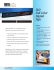### File### uv led curing products phoseon advantages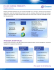### Instruction Manual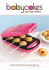### CUSTOMER SATISFACTION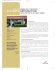### educational toolkit - American Repertory Theater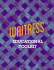### Summoning Adam: The Men`s Issue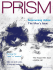### Field Expansion for Homonymous Hemianopia by Optically Induced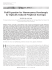### Issue 18 - Baking Industry Association of New Zealand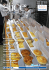### March 2013 - Ute Country News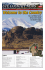### Pope - Paving Stringless with 3D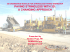### Happy Scrappy Houses!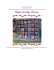### The effect of compliant prisms on subduction zone earthquakes and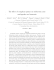### Name each polygon by its number of sides. Then classify it as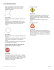### Product Guide 14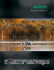### Product Guide 13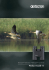### Product Catalogue 2014 EU LR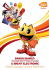### plain PDF file - Raleigh Downtowner Magazine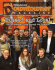### PPE, Workplace Signs and Drug Testing Catalogue (4mb pdf)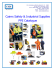### Lesson 21: Ptolemy`s Theorem